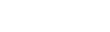# I’ve been learning JavaScript for two years now and got familiar with almost all of its tricks pretty soon — the map function, filter, sort, and others.Shivam Bhasin

4 months ago | 4 min read
FollowI’ve been learning JavaScript for two years now and got familiar with almost all of its tricks pretty soon — the map function, filter, sort, and others.

Well, not lying, I got stuck at points but some decent explanations were covering these topics on the web or YouTube except for this one thing —the reduce method.

And then I wondered why no one was talking about the reduce method confidently like other methods in JavaScript.

This led me to dig deep into it and figure out the what, why, and how of the reduce method in JavaScript. After this 4 min read, the one thing that I guarantee you is you’ll be using the reduce method in your code more confidently.

## So what is it?

Mozilla docs define the reduce method as,

"The reduce() method executes a user-supplied “reducer” callback function on each element of the array, passing in the return value from the calculation on the preceding element. The final result of running the reducer across all elements of the array is a single value"

That seems scary at first but let’s simplify this in layman terms. Here’s my definition of it.

"The reduce() method traverses the array from left to right and for each element it calculates the next value given an existing value and a method to calculate the new value. The existing value is the intial value for first iteration and result of previous iteration for all other iterations. It returns the value of the final iteration as the result"

I hope this one’s clearer. The syntax for the reduce method is,

``arr.reduce(   (accumulator, currentValue, index, array) => {}, // callback function    initialValue )``

Now that you’ve seen the syntax, let’s break down my definition of the reduce method even further.

• It will traverse through all the elements inside the array just like the map or filter methods.
• It will calculate a value for each iteration based on the method we provide it. Again, same as map or filter methods. This method is what we call the callback function.
• For each iteration, we will get the result of the previous iteration in the callback function. This result will be in the first argument of the callback function, the accumulator.
• In the case of the first iteration, this value will be the initialValue what we provide as a second argument to the reduce method.
• The second argument of the callback function is the current element of the array for which this iteration is going. This again is similar to what we get as the first argument in the callback for the map method.
• The result of the last iteration is what we get out of the reduce method. One thing to pay attention to here is that reduce returns a single value. We get a single value out of the reduce method in JavaScript, unlike the map or filter methods.
• index and array are optional parameters where the index is the index of the current iteration’s element just like the map function and the array is the complete array on which we are using the reduce method.

Wooh! That was pretty much the what of the reduce method of JavaScript. Pat yourself on the back, you’ve made it till here.

So now we know what the reduce method is. Let’s see how we can use it to write a neat and clean JavaScript code.

## Why reduce?

We can do refactor a lot of code with our knowledge of the reduce method. Let’s start with a simple example. Let’s suppose we need to find the sum of all elements of an array. Before the knowledge of the reduce method, here’s how I’d have done it.

``const arr = [1,2,3,4,5];let sum = 0;for (let i = 0; i < arr.length; i++) {     sum += arr[i]; } console.log(sum); // 15``

But that looks childish. This doesn’t look like a modern-day JavaScript code, it’s a for loop. Here’s how I’m doing it now.

``const sum = [1,2,3,4,5].reduce((a,c)=>a+c, 0);console.log(sum); // 15``

Now that looks like JavaScript. Also, notice how in the for loop code we used let for variable sum, but with the reduce method, we can use const.

Let’s simplify this a bit more. Our callback function is (a,c) => a+c , which is pretty much straightforward. For every iteration, we add the current element, c of the array to the result of the previous iteration, a. Also, our initial value is 0, that is simply because the sum of no numbers taken is 0.

Okay so now I know how to get the sum of elements of an array using the reduce method. Let’s take one more step towards reduce and calculate the average of all elements of an array. I’m not telling you the for loop solution to this, because you’re smart enough if you’ve stayed till here. Let’s see the reduce solution to this.

``[10,20,10,5,5].reduce((a,c,i) => {     a+=c;     if(i===4) return a/5;      else return a;}); // 10``

In the above example, I added the current element to the accumulator and then checked whether this is the last iteration. If yes, I returned the sum divided by the size of the array. Else, I’ve returned the sum.

Here’s a parting tip if you’ve stayed this long. Notice that in the above example I’ve not given an initial value to my reduce method. It is an optional parameter and if not provided the first element of the array is taken as the initial value and iterations start from the second element.

That was pretty much about the reduce method in JavaScript. Hope you’ve learned something new today. Do check this space for more JavaScript’y things.

Let the geek inside you win!

More content at plainenglish.io

UpvoteCreated by

Shivam Bhasin

Follow

Engineer | Learner | Writer

Simplifying things since childhood. Writes about JavaScript, application development, and self-help.Post

Upvote

Downvote

Comment

Bookmark

Share

Related Articles